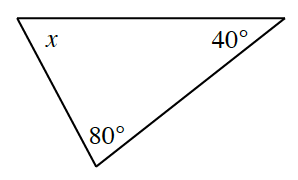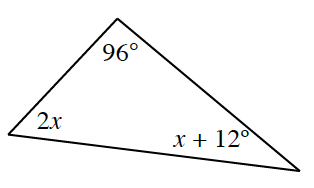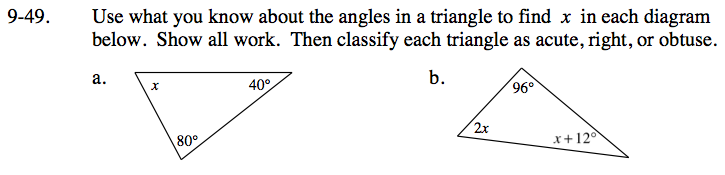### Home > CC3 > Chapter 9 > Lesson 9.1.4 > Problem9-49

9-49.
1. Use what you know about the angles in a triangle to find x in each diagram below. Show all work. Then classify each triangle as acute, right, or obtuse. Homework Help ✎

1.2.By the Angle Sum Theorem, the sum of all interior angles of a triangle is always 180°.

x + 40 + 80 = 180

x + 120 = 180

x = 60°

(2x) + (x + 12) + (96) = 180